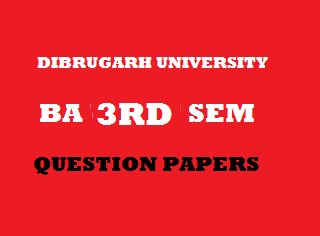## Thursday, January 03, 20192014
(November)
COMPUTER SKILL
(For B.A. Major and General/For B.SC. General)
Course: 301
[PC-Software]
(Group – A)
Full Marks: 48
Pass Marks: 19
Time: 2 hours
The figures in the margin indicate full marks for the questions

1. Answer the following as directed: 1x5=5
1. What is desktop?
2. Round (89.642) will return ____. (Fill in the blank)
3. It is impossible to copy a formula in MS-Excel.  (State True or False)
4. ____ is the extension of a PowerPoint file. (Fill in the blank)
5. What is filtering?
2. (a) What is a desktop shortcut? 2
(b) Write the steps to save a workbook in MS-Excel. 2
(c) What are the four slide layouts available in PowerPoint? 2
(d) Write down the steps to add header and footer in an MS-word document. 2
(e) What are the different types of chart available in Excel? 2
3. (a) Differentiate between slide sorter view and slide show view. 3
(b) Write the functions of operating system. 3
(c) Explain the following functions: 5
1. SUM()
2. ABS()
3. MIN()
4. MAX()
5. FLOOR()
Or
Explain in brief the features of Windows operating system. 11

4. (a) Explain the following: 3+3=6
2. Goal seek and scenario.
Or
Explain in brief about page formatting in MS-Word. 11
5. (a) On the basis of the following worksheet, answer the questions below:
 A B C D Item Price Quantity Value 12345 XYSTotalMax Price 100020003000 180300400

1. Write the formula to display ‘value’ for each item in column D. 2
2. Write the formula to display total of value in cell D4. 2
3. Write the formula to display maximum price in cell B5. 2
(b) Write the steps to perform the following in a slide of PowerPoint presentation: 5
1. Apply animation effect to each main point and sub-point.
2. Hide a slide from slide show.
3. Apply sound effect in each slide.
4. Apply design template.
5. Insert footer in a slide.
Or
Write short notes on the following: 6+5=11
1. Features of MS-PowerPoint.
2. Features of MS-Excel.

***